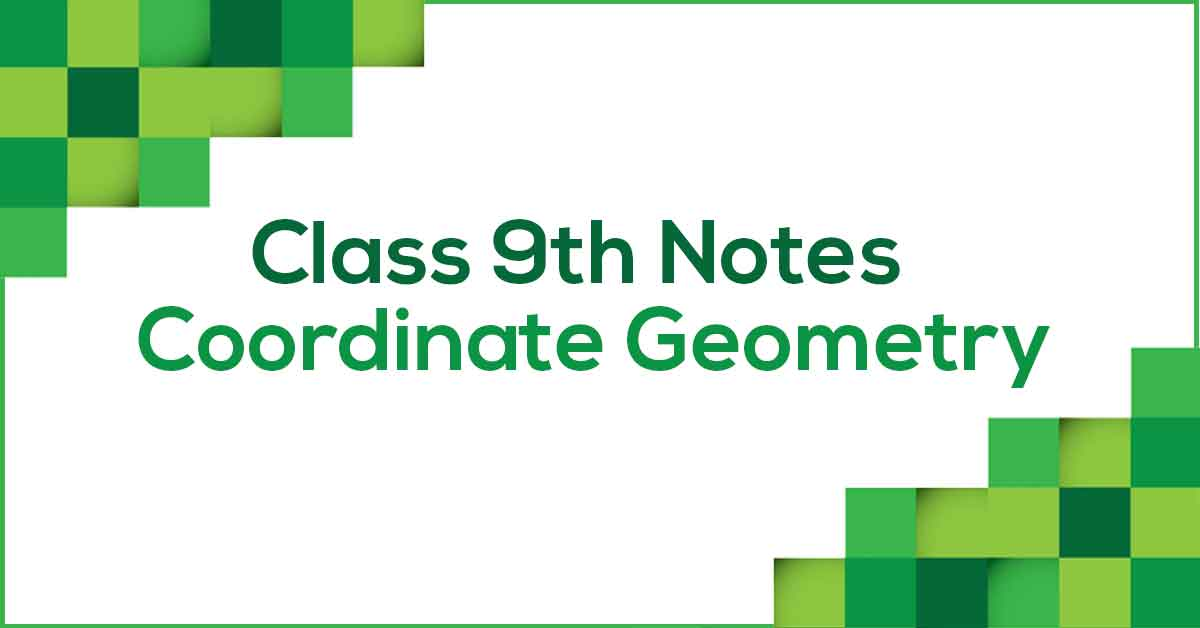Coordinate Geometry Class 9 Notes | Vidyakul
×

# Coordinate Geometry Class 9 Notes## Class 9 Maths Chapter 3 Coordinate Geometry Notes - PDF Download

Coordinate Geometry Class 9 Notes are prepared strictly according to the NCERT Syllabus which helps to get rid of any confusion among children regarding the course content since CBSE keeps on updating the course every year. These notes are prepared by our panel of highly experienced teachers which not only reduces the pressure on the students but also offer them a simple way to study or revise the chapter. Coordinate Geometry Class 9 Notes would provide you with an effective preparation for your examinations.

### How do you define Coordinate Geometry?

A coordinate geometry is a branch of geometry where the position of the points on the plane is defined with the help of an ordered pair of numbers also known as coordinates. The number line which is also known as Cartesian plane is divided into four quadrants by two axes perpendicular to each other, labeled as the x-axis (horizontal) and the y-axis (vertical line). The point at which the axes intersect is known as the origin. The location of any point on a plane is expressed by a pair of values (x, y) and these pairs are known as the coordinates.